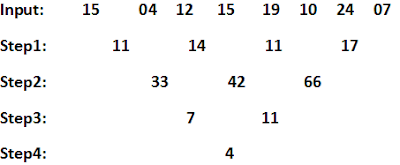## Reasoning Ability Practice Questions for IBPS Exams 2017 (11 – 10 – 2017)

Reasoning Ability Practice Questions for IBPS Exams 2017 (11 – 10 – 2017)
Directions (1 – 2): Study the following information to answer the given questions.
A word and number arrangement machine when given an input line of words and numbers rearranges them following a particular rule. The following is an illustration of input and rearrangement.

Step 4 is the last step of the rearrangement. As per the rules followed in the above steps, find out in each of the following questions.
Input: 15          04     12      15       19     10     24     07
1. What is Difference between sum of numbers of step 1 and product of numbers of step 3?
(a)  Multiple of 5
(b)  Multiple of only 3
(c)  Multiple of only 4
(d)  Multiple of both 3 and 4
(e)  Multiple of 9
2. Which number will appear in step four of given input?
(a)  6
(b)  4
(c)  3
(d)  5
(e)  8
Directions (3 – 5): Read the following information carefully and answer the questions which follow:
P, Q, R, S, T and V live on different floors in the same building having six floors numbered one to six (the ground floor is numbered 1, the floor above it, number 2 and so on and the top-most floor is number 6.)
Q lives on an even number floor. Only two people live between the floors on which Q and V live. S lives on a floor immediately above the floor on which R lives. S does not live on an odd numbered floor. P does not live on a floor which is immediately above or immediately below the floor on which T lives.
3. Who amongst the following live on the floors exactly between the floors on which Q and V live?
(a)  R, S
(b)  P, R
(c)  S, T
(d)  P, T
(e)  Cannot be determined.
4. On which of the following floors does T live?
(a)  3rd
(b)  5th
(c)  1st
(d)  4th
(e)  Cannot be determined
5. How many people live on the floors above the floor on which P lives?
(a)  None
(b)  One
(c)  Two
(d)  Three
(e)  Cannot be determined
Directions (6 – 10): Study the following information and answer the questions given below it.
Seven members H, I, J, K, L, M and N are working in different cities Ahmedabad, Bangalore, Chennai, Hyderabad, Kolkata, Delhi and Mumbai not necessarily in the same order. Each one has a different mother tongue Tamil, Kannada, Telugu, Hindi, Marathi, Punjabi and Bangla not necessarily in the same order.
J works in Bangalore and his mother tongue is not Tamil or Marathi. K’s mother tongue is Punjabi and he works in Ahmedabad. L and M do not work in Chennai and none of them has Marathi mother tongue. I works in Hyderabad and his mother tongue is Telugu. The one who works in Delhi has Bangla mother tongue. N works in Mumbai and his mother tongue is Hindi. L does not work in Kolkata.
6. Who works in Delhi?
(a)  H
(b)  M
(c)  L
(d)  K
(e)  None of these
7. What is L’s mother tongue?
(a)  Bangla
(b)  Marathi
(c)  Telugu
(d)  Cannot be determined
(e)  None of these
8. What is H’s mother tongue?
(a)  Telugu
(b)  Hindi
(c)  Bangla
(d)  Marathi
(e)  None of these
9. Who works in Kolkata?
(a)  H
(b)  L
(c)  M
(d)  L or M
(e)  None of these
10. Which of the following combination is correct?
(a)  Marathi – I – Hyderabad
(b)  Tamil – M – Kolkata
(c)  Marathi – I – Chennai
(d)  Punjabi – K – Delhi
(e)  None of these

Solutions:
(1 – 2): Explanation:
Here mathematical operation is followed in every step
Input: 15    11     18     14     16     06     19     13
Average of first and last and second and second last number and so on taken and written.
Step1: (15 + 13)/2 = 14   (11 + 19)/2 = 15  (18 + 06)/2 = 12  (14 + 16)/2 = 15
First number is multiplied with with difference with his next number
Step2: 14 × (15 – 14) = 14   15 × (15 – 12) = 45      12 × (15 – 12) = 36
Second number is divided by sum of digit of his previous number
Step3: 45/(1 + 4) = 45/5 = 9 36/(4 + 5) = 36/9 = 4
Difference between numbers
Step4: 9 – 4 = 5
Now, for Input:1. D) Sum of numbers of step 1  11 + 14 + 11 + 17 = 53
Product of numbers of step 3 7 × 11 = 77
Difference = 77 – 53 = 24
As we can see 24 is multiple of both 3 and 4 therefore this is correct option.

2. B)

(3 – 5): Explanation:
 Floor No. Person 6 P/T 5 V 4 S 3 R 2 Q 1 T/P
3. A)   4. E)   5. E)
(6 – 10): Explanation:
 Person City Mother Tongue H Chennai Marathi I Hyderabad Telugu J Bangalore Kannada K Ahmedabad Punjabi L Delhi Bangla M Kolkata Tamil N Mumbai Hindi
6. C)   7. A)   8. D)   9. C)   10. B)